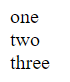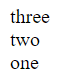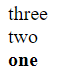# do_action and add_action in Woocommerce and WordPress

It’s quite hard to give an easy to understand definition of what do_action and add_action do, so I’m just going to launch straight into some code and hopefully, the purpose of the two methods will become clear reasonably quickly. Here’s a very simple example of do_action

`do_action("display_some_numbers");`

If we add the code above to a page then absolutely nothing will happen, so to move things along a bit lets define a function that outputs a number.

```function OutputOne() {
echo "one<br>";
}```

Now we have a function we can link it to our do_action call using the code below

```function OutputOne() {
echo "one<br>";
}

do_action("display_some_numbers");```

Now the magic is starting to happen, the script above should now output “one”.

So what has gone on here? When we call do_action we pass a tag to it, in our case we pass the tag “display_some_numbers”, the code then checks if any functions are linked to the tag and, if it finds any linked functions it executes them. We link functions to the tag using the  add_action method, let’s take a look at that line again.

`add_action("display_some_numbers","OutputOne");`

This line links the OutputOne  function we defined earlier to the “display_some_numbers” tag, then when we call do_action(“display_some_numbers”), the OutputOne function is called and it outputs the text “one” to the screen.

Just to further emphasize what’s happening, if we add another couple of functions similar to OutputOne and we change the code to call the add_action function three times linking both functions to the “display_some_numbers” tag

```function OutputOne() {
echo "one<br>";
}

function OutputTwo() {
echo "two<br>";
}

function OutputThree() {
echo "three<br>";
}

do_action("display_some_numbers");```

then we will get the following result## Changing the order the functions are called in

If we wanted to display the numbers in reverse order (three, two, one), then we could just change the order in which the add_action calls appear in the code, but we can also pass a third argument to add_action which specifies the order in which the functions will be called. The functions linked to the lowest numbers will be called first, so if we change our code as below

```function OutputOne() {
echo "one<br>";
}

function OutputTwo() {
echo "two<br>";
}

function OutputThree() {
echo "three<br>";
}

do_action("display_some_numbers");```

Then we wil see the output below.## Passing arguments to functions

Let’s change our OutputOne function so it accepts a couple of arguments that can make the displayed text bold and/or italic

```function OutputOne(\$isbold,\$isitalic) {
\$text = "one";

if (\$isbold) {
\$text = "<strong>".\$text."</strong>";
}

if (\$isitalic) {
\$text = "<em>".\$text."</em>";
}

echo \$text."<br>";
}```

How can we pass arguments to the functions we pass into the add_action calls? We can pass the arguments into the do_action call as below

`do_action("display_some_numbers",true,true);`

Note that even though only the OutputOne function takes any arguments, passing the arguments in to do_action has no adverse effects on the other two functions, they just ignore the arguments. Having passed the arguments in we might expect the text “one” to be shown in bold, italic font, but if we run the code the text only appears in boldWhy has this happened? Well, by default, any functions passed as an argument to the add_action function only get one parameter value passed to them. If we want to pass more arguments then we need to explicitly state that in the add_action call, as below

```//explicity state that the OutputOne function should have two arguments passed to it

do_action("display_some_numbers",true,true);```

Then the numbers are displayed as expected

## Removing functions with remove_action

Let’s say we no longer want to display the number “two” in the list, here’s how we could do that

`remove_action("display_some_numbers",OutputTwo,2)`

It’s worth noting that if you have supplied a priority/order argument when adding the function with the add_action then you must supply the same argument when removing the function.

Using the example above, if we did not supply the order argument then the function would not be removed and the value”two” would still be output.

Just as a final point on this, the remove_action function returns a boolean value so you can use that to check if your function has been successfully removed or not.

## Why bother with do_action and add_action couldn’t we just write stuff directly to the page?

This is a good question, and if all we wanted to do was write three numbers to the page then using do_action and add_action would definitely be a case of over-engineering.

do_action and add_action are however incredibly useful when we want to add and remove functionality from code without getting involved in the nitty gritty of the code itself. For example, imagine we have the following in a word press theme file.

```add_action("display_header","DisplayTitle",1);